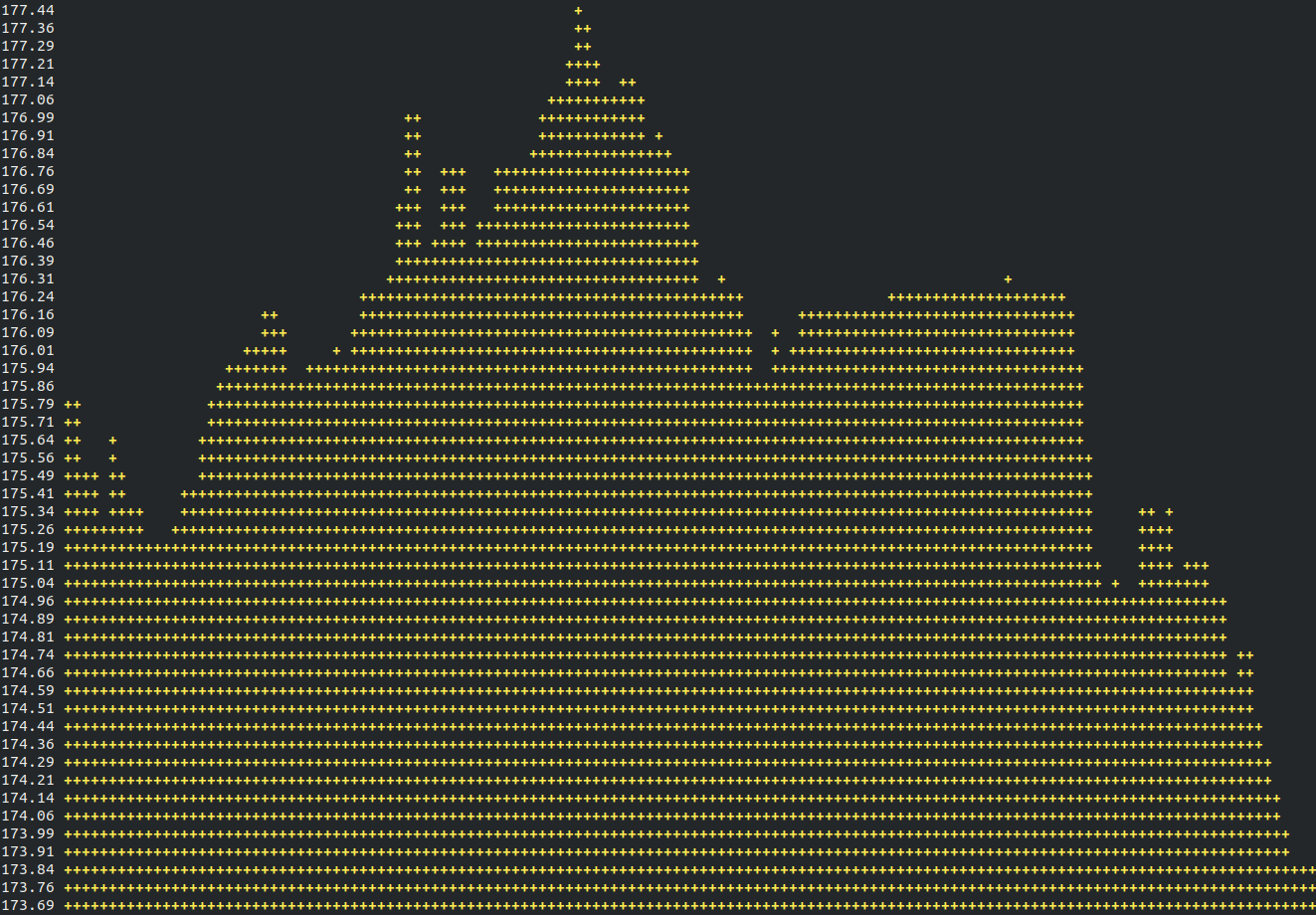# termchart Release 0.0.4

A tool to draw ascii line chart in terminal

Keywords
terminal, line, chart, graph, ascii, line-chart, python3
MIT
Install
``` pip install termchart==0.0.4 ```

### TermChart

Draw ascii line charts in terminal.### Install

`pip3 install termchart`

### Usage

Create a Python file :

```import termchart

graph = termchart.Graph([1,2,3,2,5,1,-1,-5,-3])
graph.draw()```

You can change the plot (default is `+`):

`graph.setDot('|')`

Change the width and height (default cols is 160x50)

```graph.setCols(200)
graph.setRows(40)```

Add values whenever you need it with `addData(<Float>)`. Here is a full example for a live graph with random values :

```import termchart
import time
import os
from random import randint

graph = termchart.Graph([])
while True:
rand = randint(0, 9)